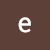# Program to add two binary numbers using bin function

Program to add two binary numbers using bin function.

A program that combines two binary numbers

In the following program, we use two built-in functions int () and bin ().

The int () function takes into account the source value provided by the given string‌, in the following example we convert the string (which is a binary value) to an integer, so we set the source value to 2 (binary numbers have base 2, decimals have a base value of 10).

After converting strings to integer values. we add them and the result is converted to binary number using bin () function.

Originally published at https://www.epythonguru.com.

--

--

# Find the Sum of the series: 1+1/2+1/3..+ 1/N in Python

In this, you will learn how to write a Python program to find the Sum of the series: 1 + 1/2 + 1/3 +… .. + 1 / N.

1. Take the number of terms to find the sum of the series.

2. Sum is initialized to zero.

3. Use a for loop to find the sum of the series and increment number by one at every iteration.

4. Print the whole series after rounding it to three decimal places.

# Let’s take a look at the code how to find the sum of series in Python Programming.

`enter number of terms:7 ['1/1', '1/2', '1/3', '1/4', '1/5', '1/6', '1/7'] sum of series is: 2.593`

Originally published at https://www.epythonguru.com.

--

--